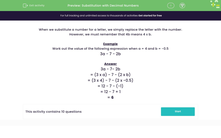# Substitution with Decimal Numbers

In this worksheet, students substitute decimal numbers for letters and work out the value of simple expressions with two variables.Key stage:  KS 3

Curriculum topic:   Algebra

Curriculum subtopic:   Substitute Numerical Values for Formulae/Expressions

Difficulty level:#### Worksheet Overview

When we substitute a number for a letter, we simply replace the letter with the number.

However, we must remember that 4b means 4 x b.

Example

Work out the value of the following expression when a = 4 and b = -0.5

3a - 7 - 2b

3a - 7- 2b

= (3 x a) - 7 - (2 x b)

= (3 x 4) - 7 - (2 x -0.5)

= 12 - 7 - (-1)

= 12 - 7 + 1

= 6

### What is EdPlace?

We're your National Curriculum aligned online education content provider helping each child succeed in English, maths and science from year 1 to GCSE. With an EdPlace account you’ll be able to track and measure progress, helping each child achieve their best. We build confidence and attainment by personalising each child’s learning at a level that suits them.

Get started